SPSS TUTORIALS BASICS ANOVA REGRESSION FACTOR CORRELATION

# Covariance – Quick Introduction

## Covariance - What is It?

A covariance is basically an unstandardized correlation. That is: a covariance is a number that indicates to what extent 2 variables are linearly related. In contrast to a (Pearson) correlation, however, a covariance depends on the scales of both variables involved as expressed by their standard deviations.

The figure below visualizes some correlations and covariances as scatterplots.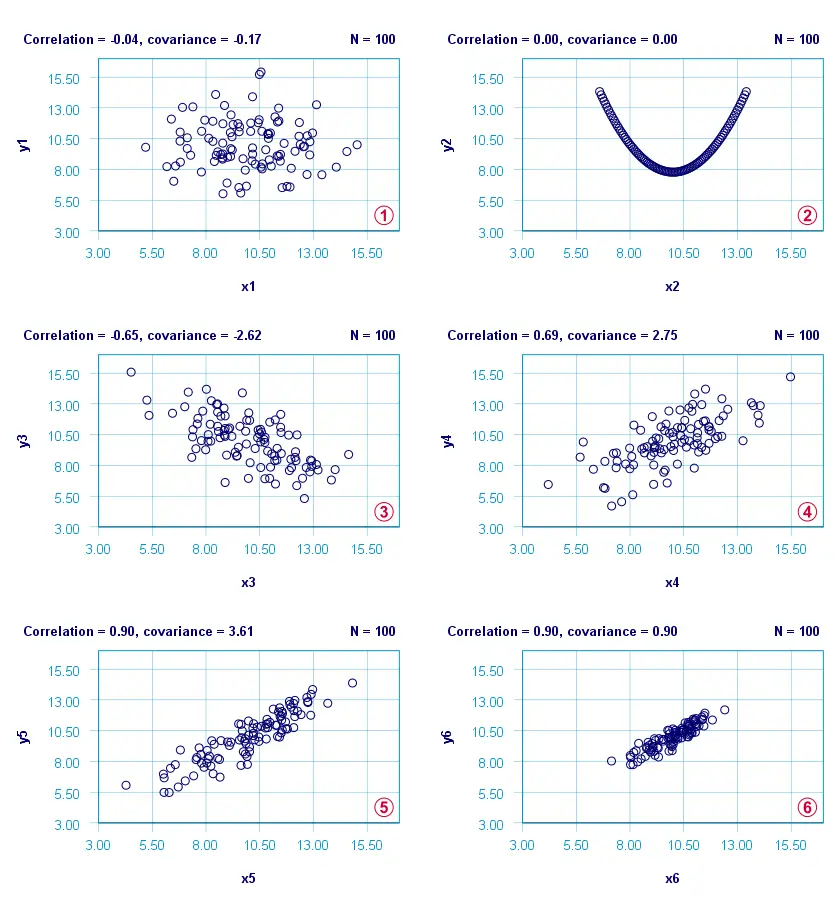x1 and y1 are basically unrelated. The covariance and correlation are both close to zero;x2 and y2 are strongly related but not linearly at all. The covariance and correlation are zero.x3 and y3 are negatively related. The covariance and correlation are both negative;x4 and y4 are positively related. The covariance and correlation are both positive;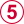x5 and y5 are strongly positively related. Because they have the same standard deviations as x4 and y4, the correlation and covariance both increase;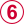x6 and y6 are identical to x5 and y5 except that their standard deviations are 1.0 instead of 2.0. This shrinks the covariance with a factor 4.0 but does not affect the correlation.

Comparing plotsandemphasizes that covariances are scale dependent whereas correlations aren't. This may make you wonder why should I ever compute a covariance

## Covariance or Correlation?

First off, the precise relation between a covariance and correlation is given by

$$S_{xy} = r_{xy} \cdot s_x \cdot s_y$$

where

• $$S_{xy}$$ denotes the (sample) covariance between variables $$X$$ and $$Y$$;
• $$r_{xy}$$ denotes the (Pearson) correlation between $$X$$ and $$Y$$;
• $$s_x$$ and $$s_y$$ denote the (sample) standard deviations of $$X$$ and $$Y$$.

This formula shows that a covariance can be seen as a correlation that's “weighted” by the product of the standard deviations of the 2 variables involved: everything else equal, larger standard deviations result in larger covariances.

This feature may be desirable for comparing associations among variable pairs. This only makes sense if all variables are measured on identical scales such as dollars, seconds or kilos. Some analyses that require covariances are the following:

1. Cronbach’s alpha is usually computed on covariances instead of correlations. This is because scale scores are computed as sums or means over unstandardized variables. Therefore, variables with larger SD's have more impact on scale scores. This is why associations among such variables also have more weight in the computation of Cronbach's alpha.

2. In factor analysis, a covariance matrix is sometimes analyzed instead of a correlation matrix. If so, associations among variables have more impact on the factor solution insofar as these variables have larger SD's.

3. Some analyses need to meet the assumption of equal covariance matrices over subpopulations. An example is MANOVA, in which the Box test -basically a multivariate expansion of Levene's test- is often used for testing this assumption.

4. Somewhat surprisingly, ANCOVA -meaning analysis of covariance- does not involve computing covariances.

So those are some analyses that involve covariances. So how are these computed? Well, which formula to use depends on which type of data you're analyzing.

## Sample Covariance Formula

If your data contain a sample from a much larger population (usually the case), the sample covariance is computed as

$$S_{xy} = \frac{\sum\limits_{i = 1}^N(X_i - \overline{X})(Y_i - \overline{Y})}{N - 1}$$

where

• $$S_{xy}$$ denotes the (sample) covariance between variables $$X$$ and $$Y$$;
• $$\overline{X}$$ and $$\overline{Y}$$ denote the sample means for $$X$$ and $$Y$$;
• $$N$$ denotes the total sample size.

Let's now get a grip on this formula by using it in a calculation example.

## Covariance Calculation Example

The table below contains the weights in grams of 10 babies at birth (X) and at age 12 months (Y). What's the covariance between X and Y?

ID12345678910
X3777327937603579413830673438405944933517
Y869578449532880795377073887311465118378604

First off,

• the sample size is $$N$$ = 10 and the means are
• $$\overline{X}$$ = 3711 and
• $$\overline{Y}$$ = 9227.

Therefore,

$$S_{xy} = \frac{(3777 - 3711)\cdot(8695 - 9227)\;+\;...\;+\;(3517 - 3711)\cdot(8604 - 9227)}{10 - 1}$$

$$S_{xy} = \frac{66 \cdot -532\;+\;...\;+\;-194 \cdot -623}{10 - 1}$$

$$S_{xy} = \frac{5189622}{10 - 1} = 576625$$

You can look up the entire calculation in this Googlesheet, partly shown below.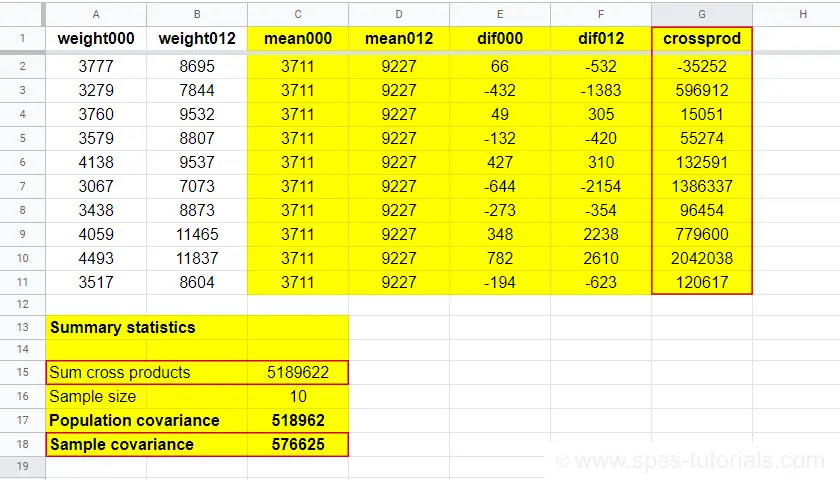## Population Covariance Formula

If your data hold the entire population you'd like to study, you can compute the covariance as

$$\sigma_{xy} = \frac{\sum\limits_{i = 1}^N(X_i - \mu_x)(Y_i - \mu_Y)}{N}$$

where

• $$\sigma_{xy}$$ denotes the (population) covariance between variables $$X$$ and $$Y$$;
• $$\mu_x$$ and $$\mu_y$$ denote the population means for $$X$$ and $$Y$$;
• $$N$$ denotes the population size.

## Software for Computing Covariances

Both sample and population covariances are easily computed in Googlesheets and Excel. This Googlesheet, partly shown below, contains a couple of examples.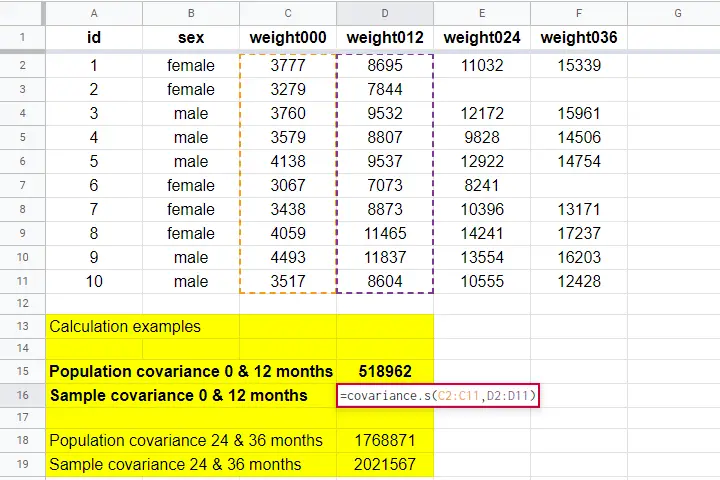A full covariance matrix for several variables is easily obtained from SPSS. However, “covariance” in SPSS always refers to the sample covariance because the population covariance is completely absent from SPSS. Pretty poor for a “statistical package”. But anyway: the only menu based option for this is AnalyzeCorrelateBivariate as illustrated below.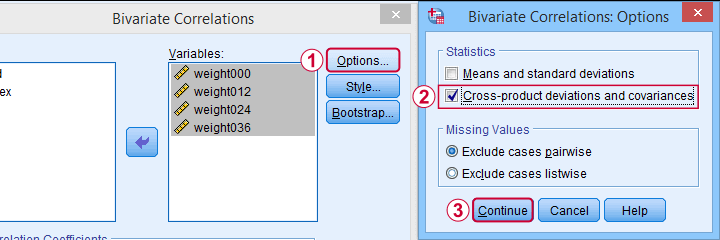A much better option, however, is using SPSS syntax like we did in Cronbach’s Alpha in SPSS. This is faster and results in a much nicer table layout as shown below.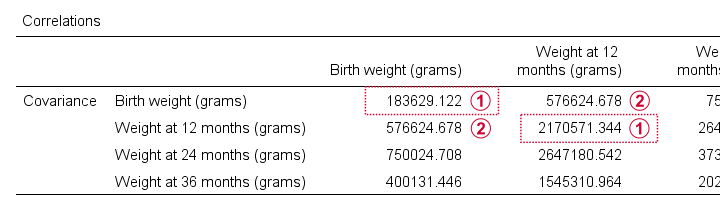Two quick notes are in place here:Just like a correlation matrix, a covariance matrix is symmetrical: the covariance between X and Y is obviously equal to that between Y and X.The main diagonal contains the covariances between each variable and itself. These are simply the variances (squared standard deviations) of our variables. This last point implies that we can compute a correlation matrix from a covariance matrix
but not reversely.
For example, the correlation between our first 2 variables is

$$r_{xy} = \frac{576625}{\sqrt{183629} \cdot \sqrt{2170571}} = 0.913$$

Right. I guess that should do regarding covariances. If you've any feedback, please throw us a comment below. Other than that:

# Tell us what you think!

*Required field. Your comment will show up after approval from a moderator.

# THIS TUTORIAL HAS 8 COMMENTS:

•### By Jon K Peck on September 23rd, 2021

The population covariance is almost never desired in my experience, and the difference from the sample covariance is miniscule with any reasonable sample size. However, it can be computed in SPSS Statistics using the MATRIX procedure. For example,

set mdisplay=table.

MATRIX.

GET data /variables weight000 weight012 /missing=omit /names=thenames.

compute data = data - make(nrow(data), 1, 1) * (csum(data) / nrow(data)).
compute sscpmat = sscp(data)/nrow(data).

print sscpmat /rnames=thenames /cnames=thenames.

end matrix.

The SET command here causes the print output to appear as a pivot table.

MATRIX is not the friendliest syntax in Statistics, but it is a powerful tool.

•### By Ruben Geert van den Berg on September 23rd, 2021

Hi Jon, that's really interesting!

They should apply something similar to the PROCESS output which -I believe- is also based on a huge MATRIX. block.

Thanks!

Ruben

•### By Jon K Peck on September 23rd, 2021

The MDISPLAY setting was introduced in Statistics 24, so it has been there for five releases by now, but few people have noticed it as far as I can tell.

I ran SET MDISPLAY=TABLE. and then ran a simple PROCESS macro specifying most of the possible output, and it produced a nice set of tables with no changes to the macro. In this case that was eleven tables.

•### By Ruben Geert van den Berg on September 24th, 2021

That sounds amazing!

I haven't covered PROCESS on my website (yet!) but I often use it when working with students.

As soon as I'll pick it up again, I'm going to give it a shot!

Another problem with PROCESS, though, is that its output is text. So numbers are not printed in floating point format. That also sucks because you can't easily adjust decimal places...

Oh, and we we can't (easily) add residuals to our dataset and/or obtain residual plots.

But let's not go into all that...

•### By Peninah Karomo on September 24th, 2021

Very clear illustration. How do you compute covariance in R